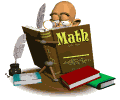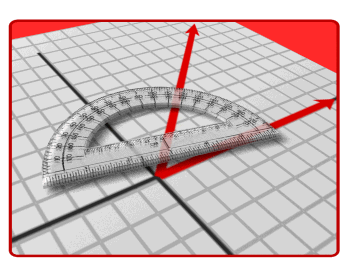• Course DescriptionAlgebra (I.II):  The branch of mathematics in which the relationship and properties of numbers are expressed and analyzed in terms of letters, numerals and abstract symbols.  Real world problems are solved using algebraic equations.

Geometry:  The branch of mathematics that deals with properties, measurements and relationships of points, lines, angles, plane figures and solids.Pre- Calculus:  The branch of mathematics that deals with trigonometric values, identities, and advanced algebra, including the study of functions.

CalculusThe branch of mathematics that deals with methods and systems of higher mathematics, the rate of which quantities change and the areas enclosed by curved boundaries.